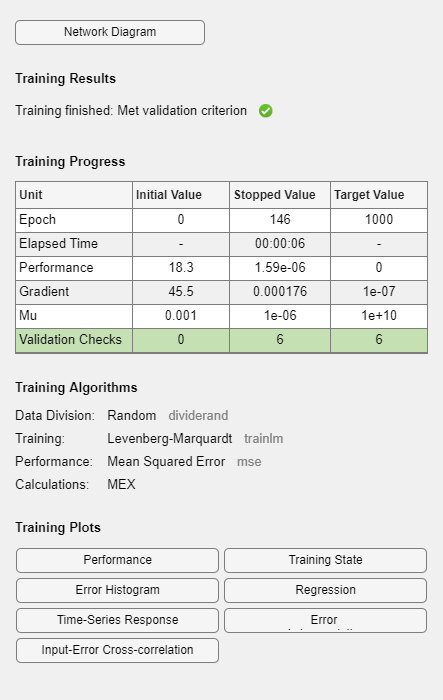Main Content

## Multistep Neural Network Prediction

### Set Up in Open-Loop Mode

Dynamic networks with feedback, such as `narxnet` and `narnet` neural networks, can be transformed between open-loop and closed-loop modes with the functions `openloop` and `closeloop`. Closed-loop networks make multistep predictions. In other words they continue to predict when external feedback is missing, by using internal feedback.

Here a neural network is trained to model the magnetic levitation system and simulated in the default open-loop mode.

```[X,T] = maglev_dataset; net = narxnet(1:2,1:2,10); [x,xi,ai,t] = preparets(net,X,{},T); net = train(net,x,t,xi,ai); y = net(x,xi,ai); view(net) ```### Multistep Closed-Loop Prediction From Initial Conditions

A neural network can also be simulated only in closed-loop form, so that given an external input series and initial conditions, the neural network performs as many predictions as the input series has time steps.

```netc = closeloop(net); view(netc) ```Here the training data is used to define the inputs `x`, and the initial input and layer delay states, `xi` and `ai`, but they can be defined to make multiple predictions for any input series and initial states.

```[x,xi,ai,t] = preparets(netc,X,{},T); yc = netc(x,xi,ai);```

### Multistep Closed-Loop Prediction Following Known Sequence

It can also be useful to simulate a trained neural network up the present with all the known values of a time-series in open-loop mode, then switch to closed-loop mode to continue the simulation for as many predictions into the future as are desired.

Just as `openloop` and `closeloop` can be used to transform between open- and closed-loop neural networks, they can convert the state of open- and closed-loop networks. Here are the full interfaces for these functions.

```[open_net,open_xi,open_ai] = openloop(closed_net,closed_xi,closed_ai); [closed_net,closed_xi,closed_ai] = closeloop(open_net,open_xi,open_ai);```

Consider the case where you might have a record of the Maglev’s behavior for 20 time steps, and you want to predict ahead for 20 more time steps.

First, define the first 20 steps of inputs and targets, representing the 20 time steps where the known output is defined by the targets `t`. With the next 20 time steps of the input are defined, use the network to predict the 20 outputs using each of its predictions feedback to help the network perform the next prediction.

```x1 = x(1:20); t1 = t(1:20); x2 = x(21:40);```

The open-loop neural network is then simulated on this data.

```[x,xi,ai,t] = preparets(net,x1,{},t1); [y1,xf,af] = net(x,xi,ai);```

Now the final input and layer states returned by the network are converted to closed-loop form along with the network. The final input states `xf` and layer states `af` of the open-loop network become the initial input states `xi` and layer states `ai` of the closed-loop network.

`[netc,xi,ai] = closeloop(net,xf,af);`

Typically use `preparets` to define initial input and layer states. Since these have already been obtained from the end of the open-loop simulation, you do not need `preparets` to continue with the 20 step predictions of the closed-loop network.

`[y2,xf,af] = netc(x2,xi,ai);`

Note that you can set `x2` to different sequences of inputs to test different scenarios for however many time steps you would like to make predictions. For example, to predict the magnetic levitation system’s behavior if 10 random inputs are used:

```x2 = num2cell(rand(1,10)); [y2,xf,af] = netc(x2,xi,ai);```

### Following Closed-Loop Simulation with Open-Loop Simulation

If after simulating the network in closed-loop form, you can continue the simulation from there in open-loop form. Here the closed-loop state is converted back to open-loop state. (You do not have to convert the network back to open-loop form as you already have the original open-loop network.)

`[~,xi,ai] = openloop(netc,xf,af);`

Now you can define continuations of the external input and open-loop feedback, and simulate the open-loop network.

```x3 = num2cell(rand(2,10)); y3 = net(x3,xi,ai);```

In this way, you can switch simulation between open-loop and closed-loop manners. One application for this is making time-series predictions of a sensor, where the last sensor value is usually known, allowing open-loop prediction of the next step. But on some occasions the sensor reading is not available, or known to be erroneous, requiring a closed-loop prediction step. The predictions can alternate between open-loop and closed-loop form, depending on the availability of the last step’s sensor reading.

Download ebook